# Improper fraction to mixed number

Here you will learn about converting improper fractions to mixed numbers, including how to recognize improper fractions and mixed numbers.

Students will first learn about converting improper fractions to mixed numbers as part of number and operations – fractions in 4th grade.

## What are improper fractions and mixed numbers?

Improper fractions and mixed numbers are both ways to show numbers that have wholes and parts. To understand improper fractions, first consider proper fractions.

A proper fraction is a fraction where the numerator (top number) is smaller than the denominator (bottom number).

For example,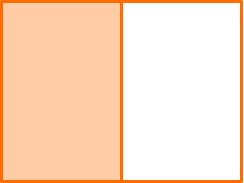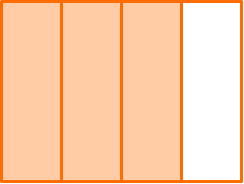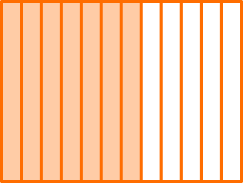\hspace{2.8cm} \cfrac{1}{2} \hspace{3.8cm} \cfrac{3}{4} \hspace{3.7cm} \cfrac{7}{12}

An improper fraction is a fraction where the numerator (top number) is larger than the denominator (bottom number).

For example,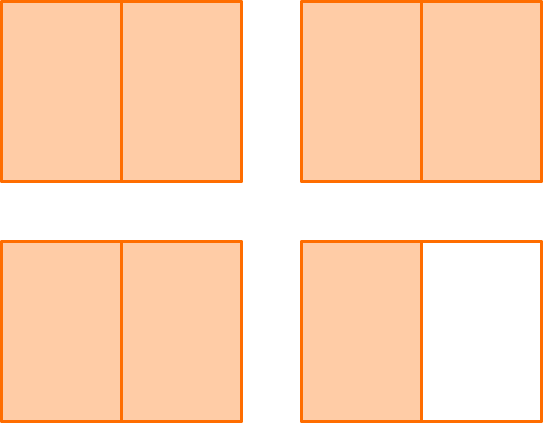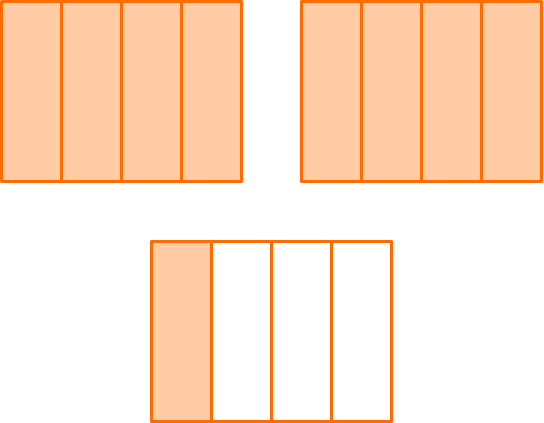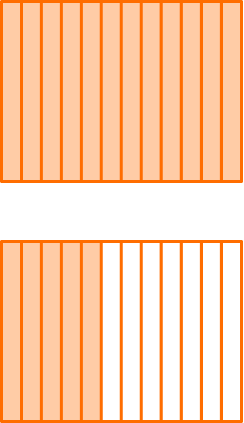\hspace{2.3cm} \cfrac{7}{2} \hspace{5.4cm} \cfrac{9}{4} \hspace{4.1cm} \cfrac{17}{12}

A mixed number has a whole number part and a fractional part

For example,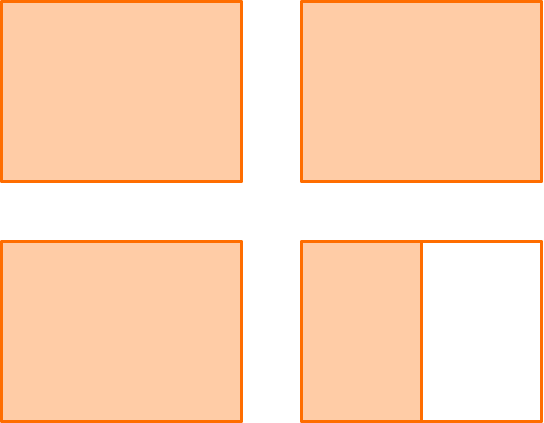\hspace{2.2cm} 3\cfrac{1}{2} \hspace{5.2cm} 2\cfrac{1}{4} \hspace{4cm} 1\cfrac{5}{12}

Any number greater than 1 can be shown as an improper fraction AND a mixed number.

For example,

\cfrac{7}{2} \, is 7 halves, which is three groups of \, \cfrac{2}{2} \, and a group of \, \cfrac{1}{2}.

3\cfrac{1}{2} \, is 3 and one half, which is three wholes and a group of \, \cfrac{1}{2}.

\cfrac{7}{2}=3 \cfrac{1}{2}

### What are improper fractions and mixed numbers?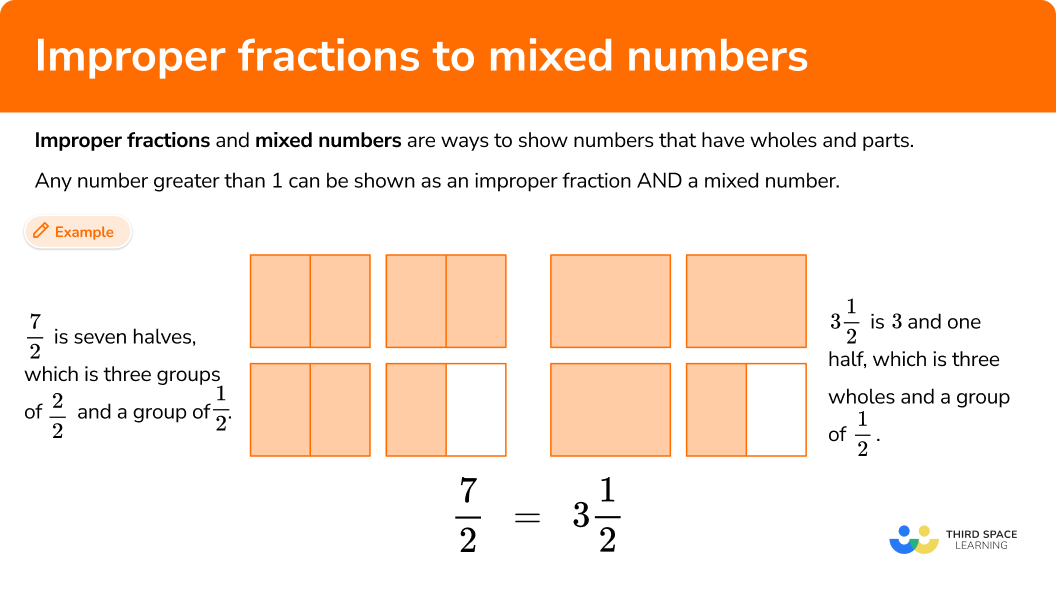## Common Core State Standards

How does this relate to 4th grade math?

• Grade 4 – Numbers and Operations – Fractions (4.NF.B.3c)
Add and subtract mixed numbers with like denominators, example, by replacing each mixed number with an equivalent fraction, and/or by using properties of operations and the relationship between addition and subtraction.

• Grade 4 – Numbers and Operations in Base 10 (4.NBT.B.6)
Find whole-number quotients and remainders with up to four-digit dividends and one-digit divisors, using strategies based on place value, the properties of operations, and/or the relationship between multiplication and division. Illustrate and explain the calculation by using equations, rectangular arrays, and/or area models.

## How to convert improper fractions to mixed numbers

In order to convert an improper fraction to a mixed number with a model:

1. Model the improper fraction.
2. Count the number of wholes and the fraction left over.
3. Write the mixed number.

In order to convert an improper fraction to a mixed number with the algorithm:

1. Divide the numerator by the denominator.
2. For the fraction, use the remainder as the numerator and keep the same denominator.
3. Write the mixed number.

## Converting improper fractions to mixed numbers examples

### Example 1: improper fraction to a mixed number with a model

Write the improper fraction \, \cfrac{7}{3} \, as a mixed number.

1. Model the improper fraction.

Draw 3 wholes and divide them equally into thirds. Then shade in 7 parts.

2Count the number of wholes and the fraction left over.

There are 2 wholes (or \cfrac{3}{3} \, ) shaded in, and there is \, \cfrac{1}{3} \, left over.

3Write the mixed number.

\cfrac{7}{3}=2\cfrac{1}{3}

### Example 2: improper fraction to a mixed number with a model

Write the improper fraction \, \cfrac{8}{5} \, as a mixed number.

Model the improper fraction.

Count the number of wholes and the fraction left over.

Write the mixed number.

### Example 3: improper fraction to a mixed number with a model

Write the improper fraction \, \cfrac{21}{6} \, as a mixed number.

Model the improper fraction.

Count the number of wholes and the fraction left over.

Write the mixed number.

### Example 4: improper fraction to a mixed number with the algorithm

Write the improper fraction \, \cfrac{42}{10} \, as a mixed number.

Divide the numerator by the denominator.

For the fraction, use the remainder as the numerator and keep the same denominator.

Write the mixed number.

### Example 5: improper fraction to a mixed number with the algorithm

Write the improper fraction \, \cfrac{17}{9} \, as a mixed number.

Divide the numerator by the denominator.

For the fraction, use the remainder as the numerator and keep the same denominator.

Write the mixed number.

### Example 6: improper fraction to a mixed number with the algorithm

Write the improper fraction \, \cfrac{51}{8} \, as a mixed number.

Divide the numerator by the denominator.

For the fraction, use the remainder as the numerator and keep the same denominator.

Write the mixed number.

### Teaching tips for converting improper fractions to mixed numbers

• In the beginning, choose worksheets that allow students a lot of space to solve. There are many different ways that students can go about converting from improper fractions to mixed numbers based on their own understanding. They can draw different types of models (including area models and number lines) or explore different operations and equations to solve. It is important that students have space to show all this thinking on paper.

• Start by letting students explore this topic through models and their own understanding of improper fractions and mixed numbers. The division algorithm becomes a logical solving strategy once students really understand how improper fractions show equal groups of the denominator.

### Easy mistakes to make

• Drawing unclear models
Models can be a useful tool to solve fractions problems, but only if they are drawn correctly. Models also tend to work better with smaller fractions. The more drawing that has to be done, the more room for error. Also consider giving students access to a digital model that creates the fraction pieces for them.

• Not keeping the denominator the same
The improper fraction and the mixed number of the same number both have the same value. This means the denominator should be the same, because the size of the parts in a fraction does not change when converting from improper fractions to mixed numbers. However, note that the denominator can change when simplifying, but this does not change the overall value of the improper fraction or mixed number.
For example,

• Dividing incorrectly
When converting, most division equations will involve quotients with remainders. Making a division error will cause the mixed number to be incorrect.

• Including an improper fraction within a mixed number
Once a number is converted to a mixed number, the fractional part should be proper. If the fraction is improper, it means that another whole can be created.
For example,
Write the improper fraction \, \cfrac{71}{9} \, as a mixed number.

### Practice improper fractions and mixed numbers questions

1. Write the following improper fraction as a mixed number: \, \cfrac{5}{4}

1\cfrac{1}{4}\cfrac{4}{5}1\cfrac{1}{5}1\cfrac{3}{4}Draw 2 wholes and divide them equally into fourths. Then shade in 5 parts.There is 1 whole (or \cfrac{4}{4} \, ) shaded in, and there is \, \cfrac{1}{4} \, left over.

\cfrac{5}{4}=1 \cfrac{1}{4}

2. Write the following improper fraction as a mixed number: \, \cfrac{8}{3}

1\cfrac{2}{3}2\cfrac{1}{3}2\cfrac{2}{3}\cfrac{3}{8}Draw 3 wholes and divide them equally into thirds. Then shade in 8 parts.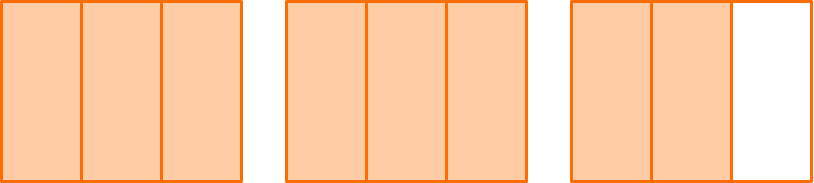There are 2 wholes (or \cfrac{3}{3} \, ) shaded in, and there is \, \cfrac{2}{3} \, left over.

\cfrac{8}{3}=2 \cfrac{2}{3}

3. Write the following improper fraction as a mixed number: \, \cfrac{23}{5}

\cfrac{5}{23}2\cfrac{3}{5}3\cfrac{4}{5}4\cfrac{3}{5}Draw 5 wholes and divide them equally into fifths. Then shade in 23 parts.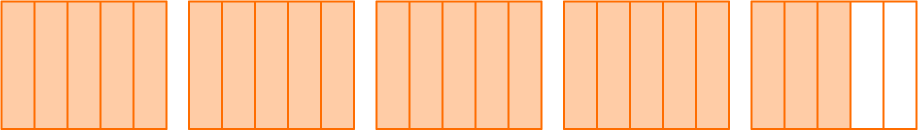There are 4 wholes (or \cfrac{5}{5} \, ) shaded in, and there is \, \cfrac{3}{5} \, left over.

\cfrac{23}{5}=4 \cfrac{3}{5}

4. Write the following improper fraction as a mixed number: \, \cfrac{43}{12}

3\cfrac{7}{12}\cfrac{12}{43}4\cfrac{1}{12}3\cfrac{1}{7}Divide the numerator by the denominator.

43 \div 12

You can use what you know about division to solve.

Since 36 + 7 = 43 and 36 \div 3=12, there will be 3 groups of 12 with 7 left over.

43 \div 12=3 \; R \, 7\cfrac{43}{12}=3 \cfrac{7}{12}

5. Write the following improper fraction as a mixed number: \, \cfrac{41}{6}

6\cfrac{6}{5}5\cfrac{5}{6}6\cfrac{5}{6}\cfrac{5}{6}Divide the numerator by the denominator.

41 \div 6

You can use what you know about subtraction to solve:

\begin{aligned} &41-6 = 35\\ &35-6 = 29\\ &29-6 = 23\\ &23-6 = 17\\ &17-6 = 11\\ &11-6 = 5 \end{aligned}

There will be 6 groups of 6 with 5 left over.

41 \div 6=6 \; R \, 5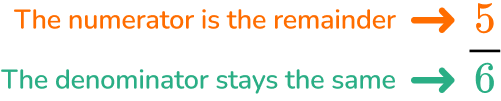\cfrac{41}{6}=6 \cfrac{5}{6 }

6. Write the following improper fraction as a mixed number: \, \cfrac{67}{5}

13\cfrac{7}{10}12\cfrac{7}{5}\cfrac{5}{67}13\cfrac{2}{5}Divide the numerator by the denominator.

67 \div 5

You can use what you know about division to solve.

Since 5 \times 12=60, then 5 \times 13=65. There will be 13 groups of 5 with 2 left over.

67 \div 5=13 \; R \, 2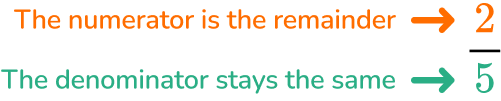\cfrac{67}{5}=13 \cfrac{2}{5}

## Improper fractions to mixed numbers FAQs

Is it possible for an improper fraction to convert to a whole number?

Yes, this is also common. For example, \frac{16}{2}=8 and \frac{55}{11}=5. Any time the numerator is a multiple of the denominator, the improper fraction will convert to a whole number without a fractional part.

Does the mixed number have to be in lowest terms?

No, students can master this skill without converting to the lowest terms (as known as the simplest form). However, there may be some instances where students are expected to record an answer in lowest terms. Check your state’s standards for specific guidelines.

What is a mixed fraction?

A mixed number is sometimes also referred to as a mixed fraction. They have the same meaning.

## Still stuck?

At Third Space Learning, we specialize in helping teachers and school leaders to provide personalized math support for more of their students through high-quality, online one-on-one math tutoring delivered by subject experts.

Each week, our tutors support thousands of students who are at risk of not meeting their grade-level expectations, and help accelerate their progress and boost their confidence.